# Input and Output Tutorial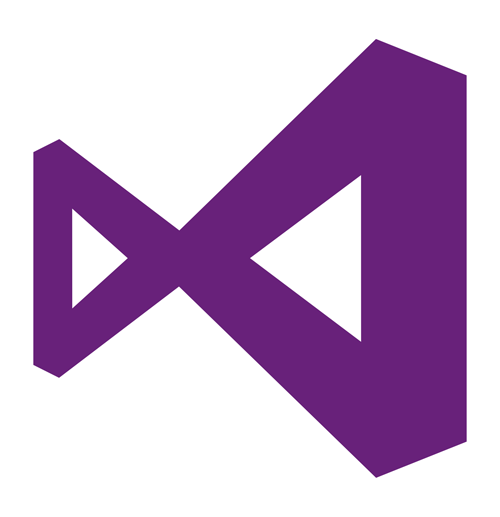Input is when the program asks you for information, and you enter it manualy. The computer will then proccess the information, then give you information or a phrase back. This is called output. For this tutorial we will be making a basic calculator. You will give the program two numbers (the input) and it will proccess that, and give you the sum (the output). Now lets learn how to do it!

1. Add the textboxes, labels and button as shown in the screenshot below. you can do this by going to the toolbox window and dragging each component onto the form.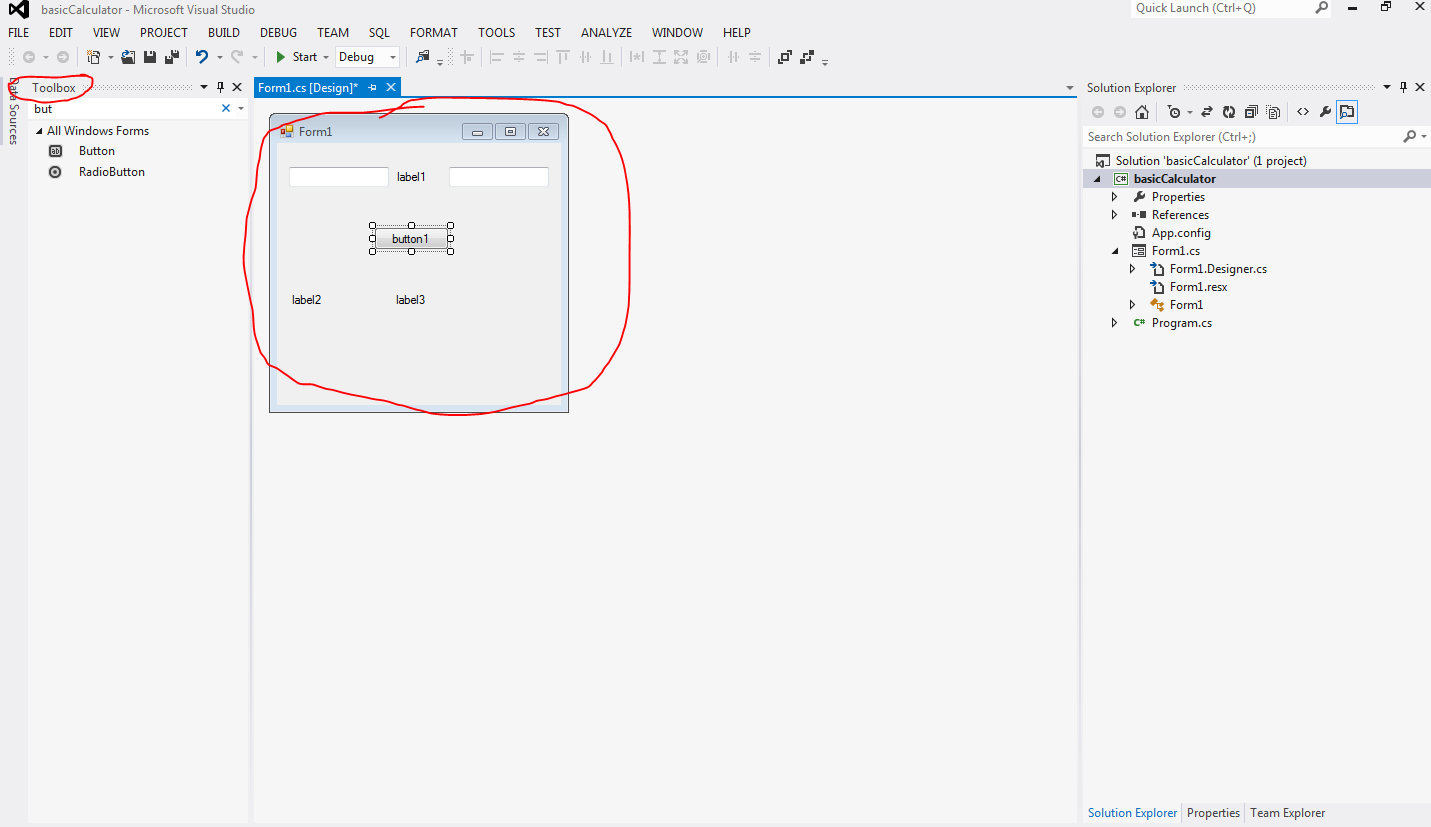2. You can change the text displayed on each of thse components by clicking on it, then going to the properties window and changing the text. You will also want to change the names of the components to something convinient so it is easier to type them out when you're coding.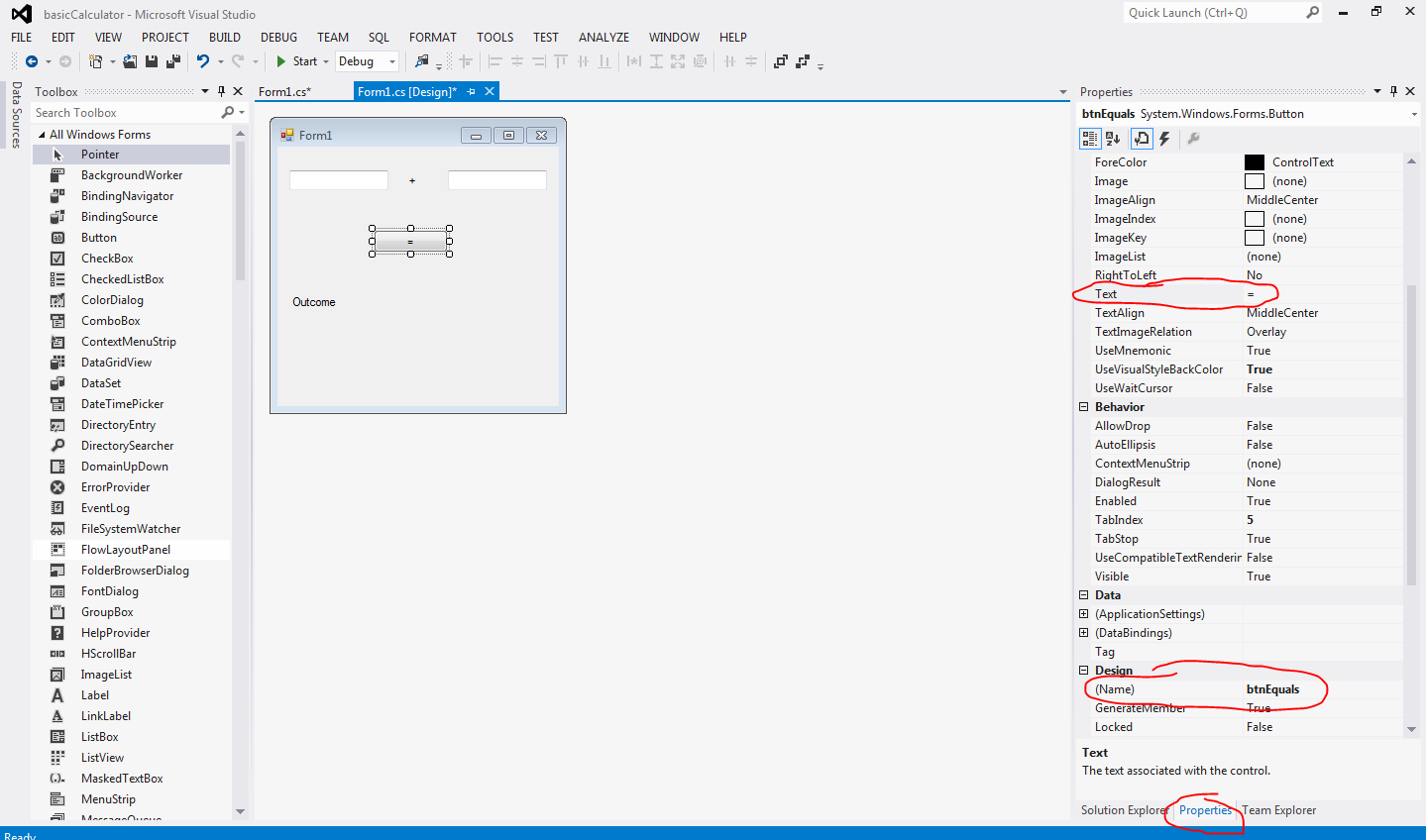3. Type the line of text highlighted in the screenshot below. What this is doing is declaring that there are going to be two numbers (the input) and an outcome (the output). Because we made it a float, The program will only be able to calculate integers. If you wanted to make a calculator that is able to calculate decimals, you would type "double" instead of "float."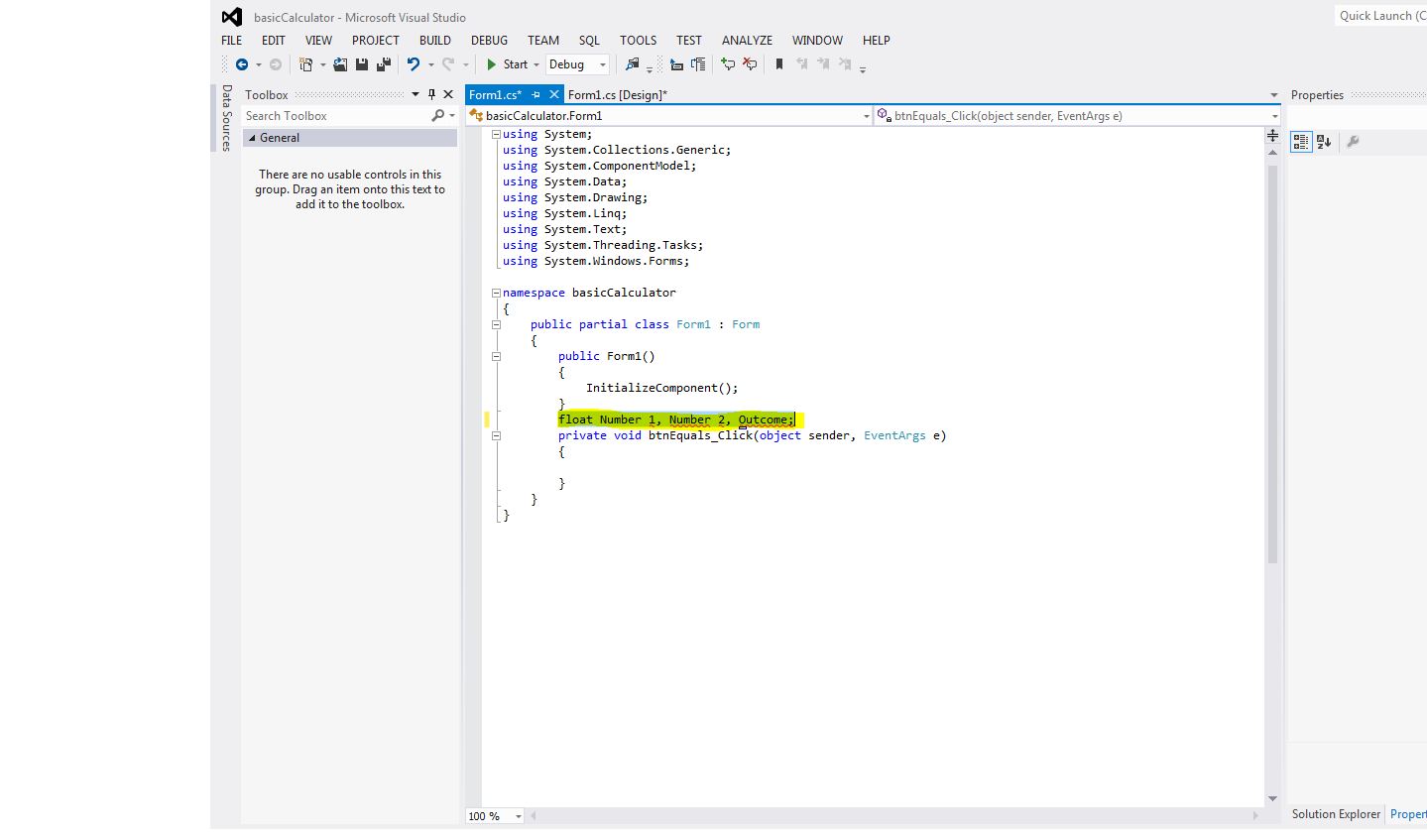4. Add the text highlighted in the screenshot below. This means that numbers 1 and 2 we declared in the float will be converted into integers (if you typed "double" instead of "float" in the previous step, you would type "ToDouble" instead of "ToInt32"). These are the numbers you will enter into the textboxes once the program is running.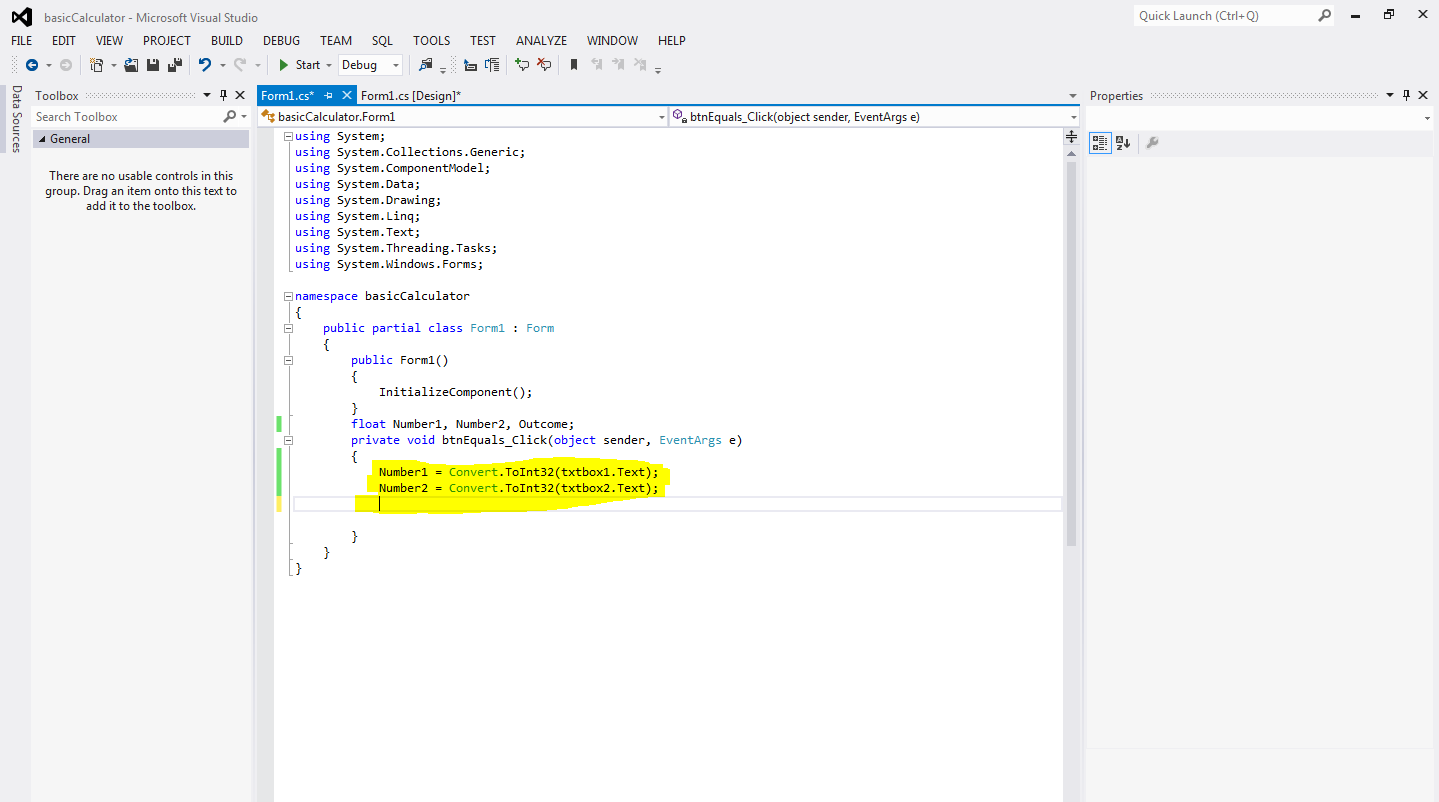5. Type the code highlighted in the screenshot below. All this means is that the outcome we declared in the float will be equal to Number1 plus Number2.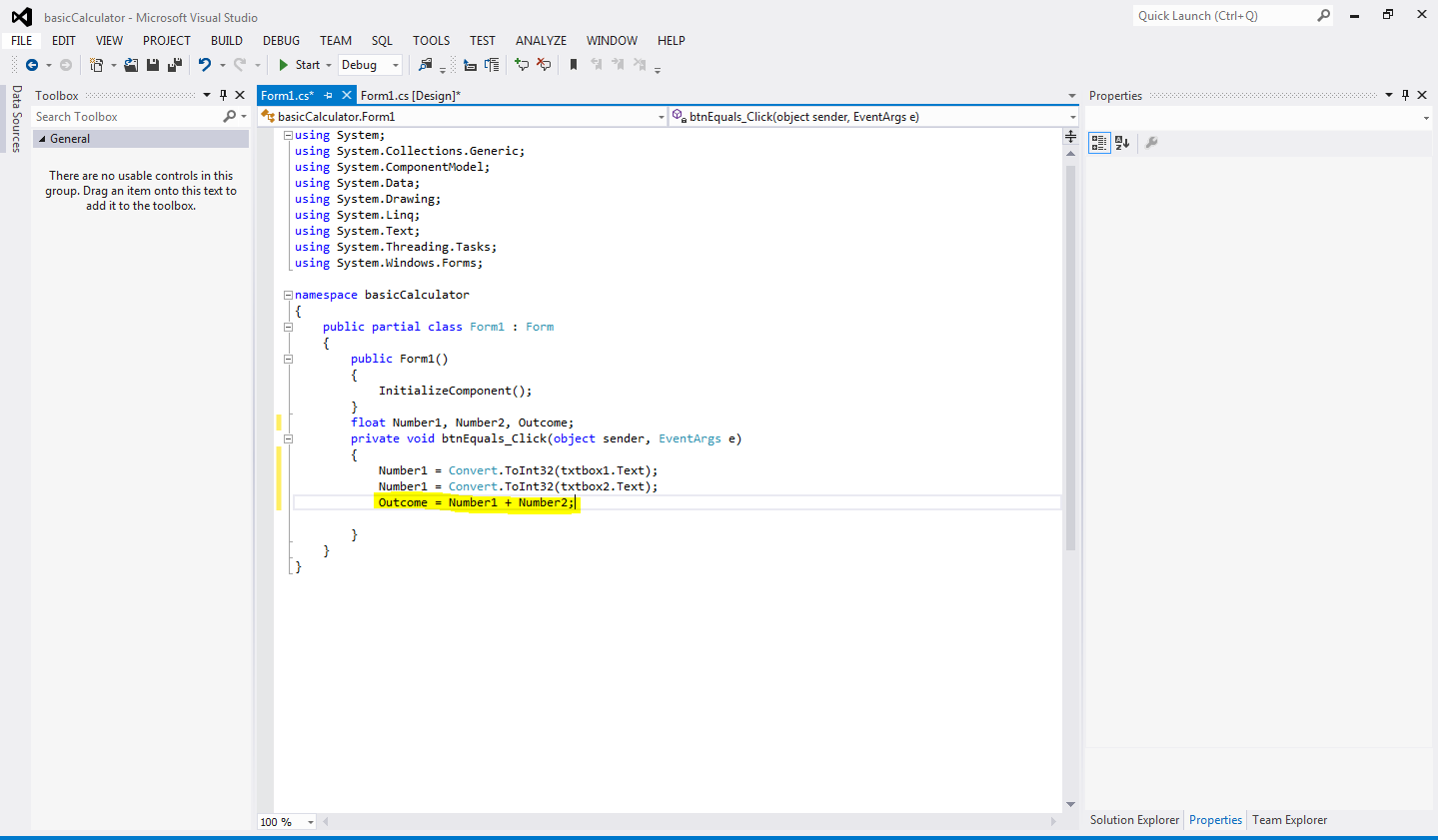6. All that is left to do is tell the program to show the outcome in the label we created at the start. This is where giving the label a convinient name comes in handy. Type the code highlighted below but remember that if you named your label something different then you will have to type that instead at the start of the line.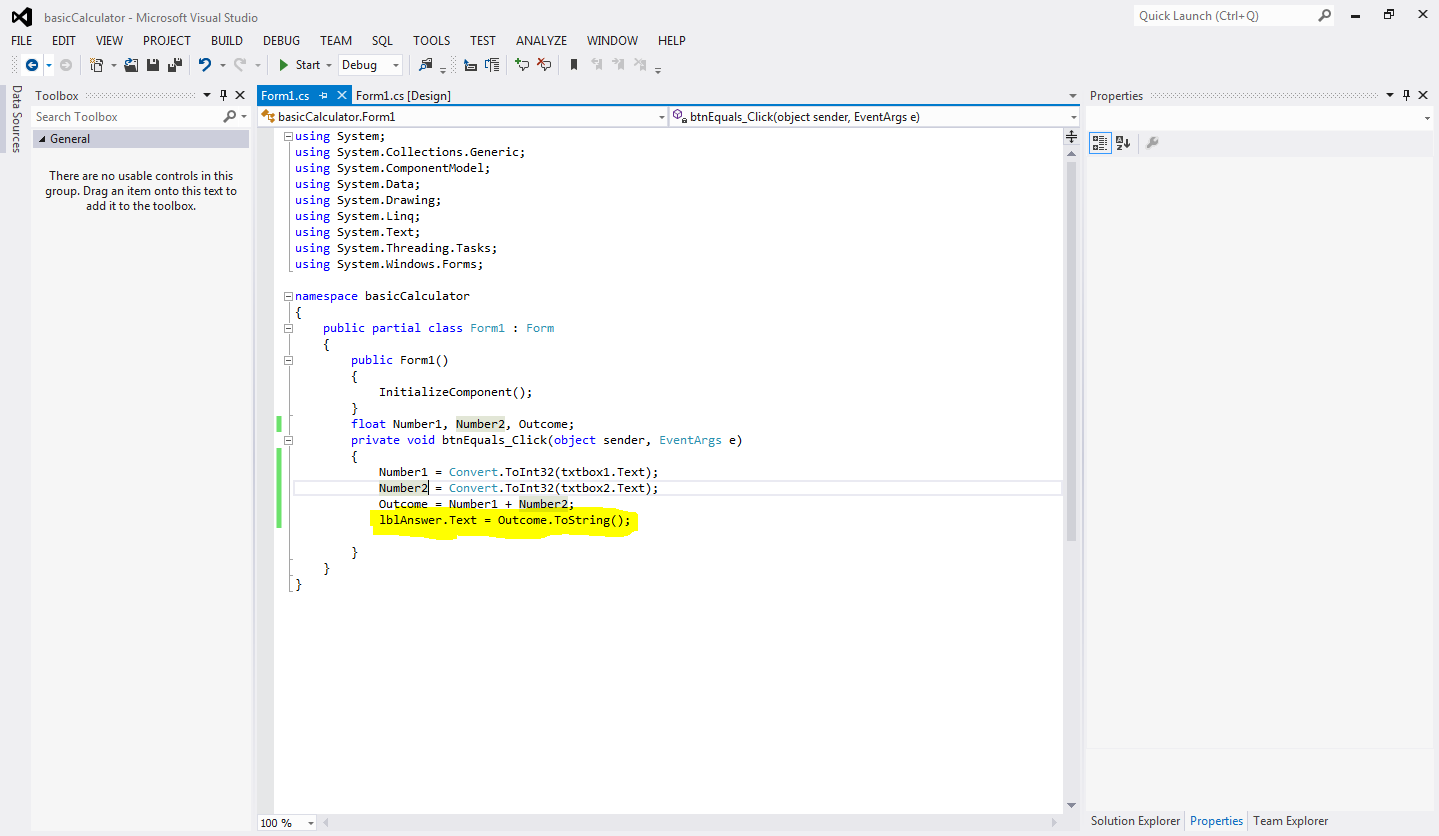7. You are now ready to run the program! hit the start button at the top of the screen and your program will pop up. Type in the two numbers you want to add together into the two textboxes and hit the "=" button. The answer will be displayed in the label below!.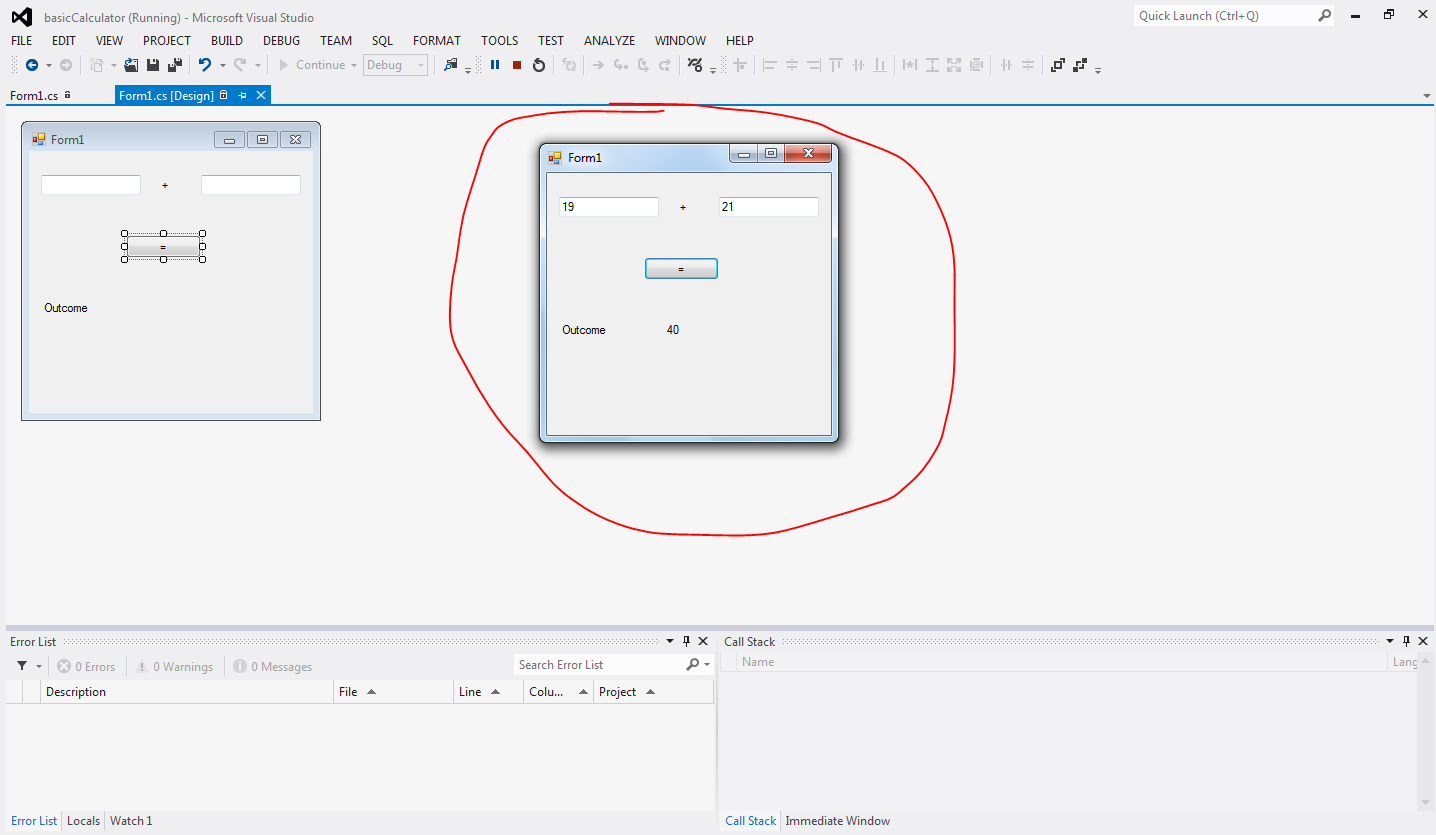Congratulations you have now finished coding your own calculator!

Go Back

Keep Learning!